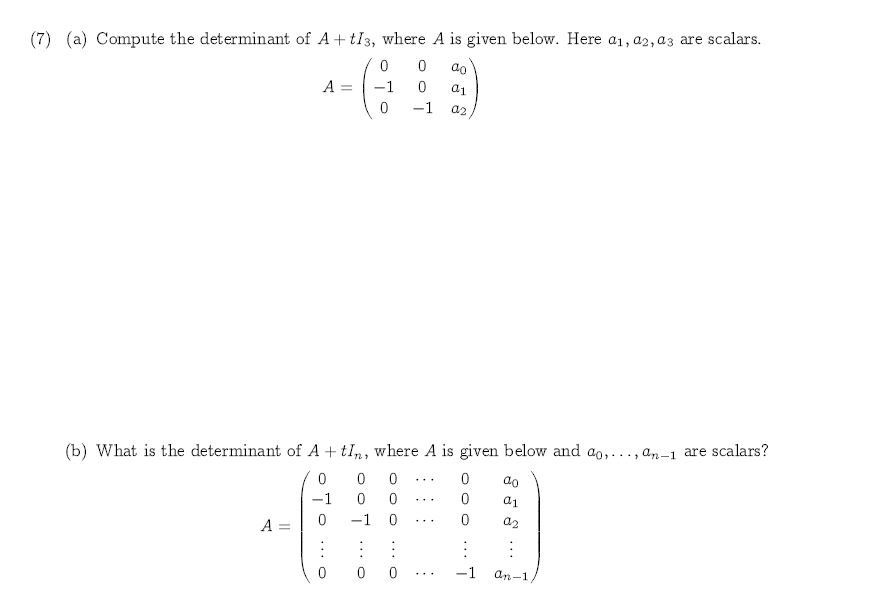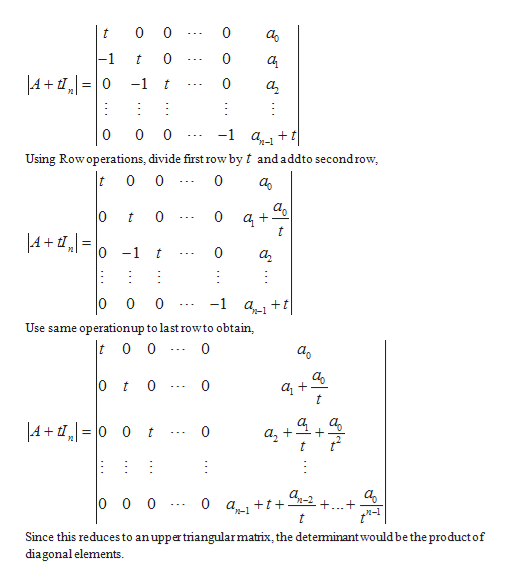# (7) (a) Compute the determinant of A tI3, where A is given below. Here a1, a2, a3 are scalars.00aoA-10-10a2(b) What is the determinant of A tIn, where A is given below and ao,. ., a-1 are scalars?0000ao-10000-100A =00 0-1an-1

Question
10 viewshelp_outlineImage Transcriptionclose(7) (a) Compute the determinant of A tI3, where A is given below. Here a1, a2, a3 are scalars. 0 0 ao A -1 0 -1 0 a2 (b) What is the determinant of A tIn, where A is given below and ao,. ., a-1 are scalars? 0 0 0 0 ao -1 0 0 0 0 -1 0 0 A = 0 0 0 -1 an-1 fullscreen
check_circle

Step 1

The determinant can be...help_outlineImage Transcriptionclose0 0 -1 0 t 0 0 t A+,0 -1 0 t 0 0 -1 at 0 Using Rowoperations, divide first row by t and addto secondrow t 0 0 0 0 0 0 t t LA+,= -1 0 t а, 0 0 0 -1 a Use same operationup to last rowto obtain, t 0 0 0 0 0 t ,A+ 0 0 I 0 + t 0 0 0 0 a t t + Since this reduces to anuppertriangular matrix, the deteminantwould be the product of diagonal elements రో ఆ fullscreen

### Want to see the full answer?

See Solution

#### Want to see this answer and more?

Solutions are written by subject experts who are available 24/7. Questions are typically answered within 1 hour.*

See Solution
*Response times may vary by subject and question.
Tagged in

### Math